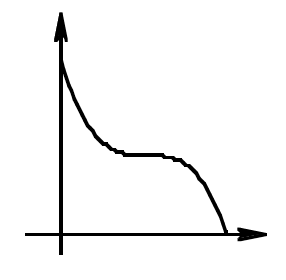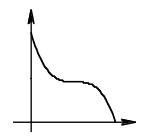### Home > CALC > Chapter 7 > Lesson 7.3.2 > Problem7-125

7-125.
1. If the graph below represents distance in miles over time between a space shuttle and Earth, how would you find the average velocity? Describe what method you would use. Homework Help ✎Given a distance function, will average velocity be represented by 'average area under the curve' or 'average rate of change'?